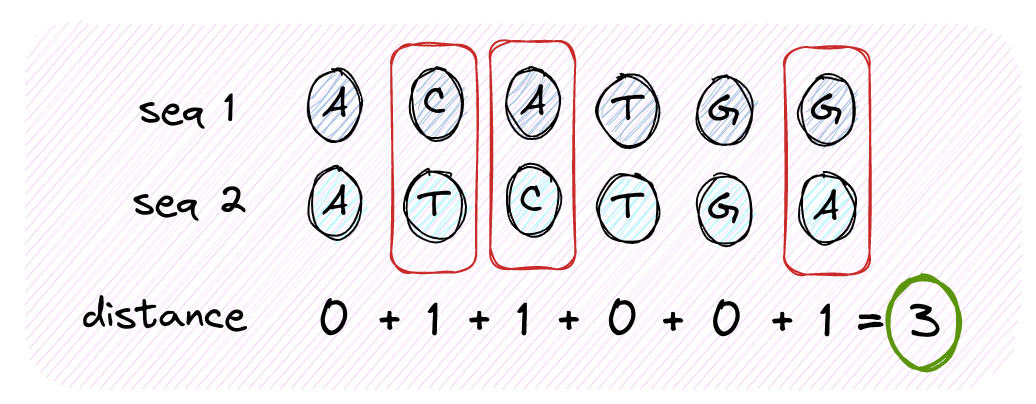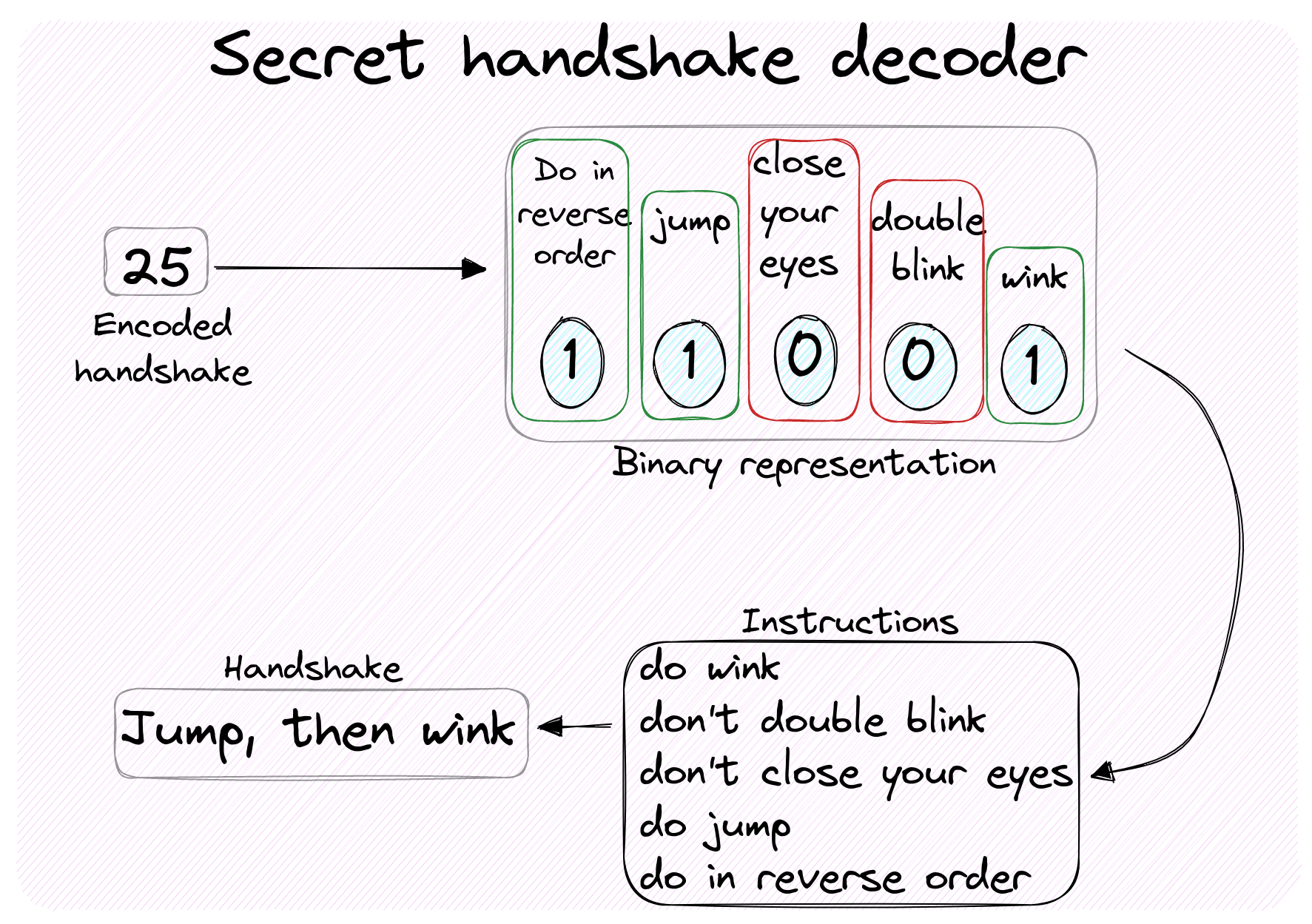## Introduction

A great feature of exercism is that once you complete an exercise, you can compare your solution with the ones other practitioners have written. This piqued my curiosity, so after I completed a few Kotlin exercises as part of the #12in#23 initiative, I went on to see how I could refactor my code to make it more idiomatic.

The following overview is what came out of that: each section corresponds to one exercise. I’ll give you a brief intro to the task, then show you my solution and finally share some refactoring tips I enjoyed.

If you recently started coding in Kotlin or if you’d like to get a sense for some of the language features in action, then you might find this helpful and discover some compelling use cases.

## Just count…Figure 1. Counting the number of differences (distance) between two DNA sequences.

In the first exercise, I was tasked to count differences across two DNA sequences - which is analogous to comparing two arrays for equality element-wise. My approach was to `zip` the two and `fold`.

``````fun compute(leftStrand: String, rightStrand: String): Int {
if (leftStrand.length != rightStrand.length) {
throw IllegalArgumentException(
"left and right strands must be of equal length")
}
return leftStrand.split("")
.zip(rightStrand.split(""))
.fold(0) {
count, (l,r) -> count + (if (l == r) {0} else {1})
}
}
``````

I found 3 improvements based on other solutions:

1. there is no need to `.split` the strings before zipping them. This is because `String` implements the `CharSequence` interface, which includes an implementation of `.zip`.
2. `fold` is always fun to use, but Kotlin offers a better alternative when the goal is to count instances satisfying a predicate: `Array#count` accepts a predicate and returns the number of instances where the predicate evaluates to `true`. This is just what we were looking for!
3. When it comes to making assertions on the parameters of a function, Kotlin offers the idiomatic `require`, which raises an `IllegalArgumentException` when the value passed is `false`.
``````fun compute(leftStrand: String, rightStrand: String): Int {
require(leftStrand.length == rightStrand.length) {
"left and right strands must be of equal length"
}

return leftStrand
.zip(rightStrand)
.count { it.first != it.second }
}
``````

Closer look. Note how convenient it is to be able to use the implicit name `it` for the lambda passed to `count`. This works anytime you have a lambda with a single argument.

## Constructor once, constructor twice

An exercise required to define a class with two 1-argument constructors accepting different types. My instinct was to define two constructors explicitly, so I wrote the following.

``````// Given a date/time, this class computes what the `LocalDateTime` 10^9 seconds in the future will be.
class Gigasecond {
val gigaSeconds = 1e9.toLong()

val date: LocalDateTime
constructor(initialDate: LocalDateTime) {
date = initialDate.plusSeconds(gigaSeconds)
}

constructor(initialDate: LocalDate) : this(initialDate.atStartOfDay())
}
``````

It turns out there is a more idiomatic option: we can use Kotlin primary constructor to save some code and remove any ambiguity around where and how the field `date` is initialised.

``````class Gigasecond(initialDate: LocalDateTime) {
val gigaSeconds = 1e9.toLong()

val date = initialDate.plusSeconds(gigaSeconds)

constructor(initialDate: LocalDate) : this(initialDate.atStartOfDay())
}
``````

Fun fact: while looking at the community solutions, I got reminded of the exponential notation as a shorthand to define large `Double` numbers. My first take in defining the `gigaSecond` constant was the unnecessarily convoluted `(10.0).pow(9).toLong()`, which among other things requires importing `kotlin.math.pow` 😅 Anyway, read on for some more `pow` fun.

Closer look. Note that I didn’t discuss the topic of class constants in this section. If you are interested, companion objects are worth a look, although some shortcomings apply.

## Circling around squaring

An exercise asked to compute the square of the sum of the first `n` natural number. It turns out there is a formula for that: `(n(n + 1) / 2)²`, so I wrote

``````fun squareOfSum(n: Int): Int {
return (n * (n + 1) / 2).toDouble().pow(2).toInt()
}
``````

I found two improvements:

1. Note how we go `toDouble` in order to invoke `Double#pow`, then have to convert back to `Int` with `toInt`. Instead, we could simply multiply the base by itself - this is a classic and recurring optimisation in code dealing with numerical computing. In other languages, we’d have to define `x = n * (n + 1) / 2`, then return `x * x`. In Kotlin, we can use `let`, which passes the receiver object as argument to a lambda and returns the result of the lambda.
2. A nice shorthand applies when a function’s body is a single expression. From the docs: “When a function returns a single expression, the curly braces can be omitted”. In that case, the body is specified after the `=` sign.
``````fun squareOfSum(n: Int): Int = (n * (n + 1) / 2).let { it * it }
``````

## Mutatis mutandisFigure 2. Decoding an integer into a sequence of signals making up a secret handshake based on its binary representation.

The next exercise asked to decipher the binary representation of an integer into a sequence of signals for a secret handshake. I came up with the following code.

``````object HandshakeCalculator {
val reverseList = 0b10000

fun calculateHandshake(number: Int): List<Signal> {
var lst = mutableListOf<Signal>()
Signal.values().forEach {
if (number.and(it.int) == it.int) { lst.add(lst.size, it) }
}
if (number.and(reverseList) == reverseList) {
return lst.reversed()
} else {
return lst
}
}
}
``````

I really like that we can iterate over the values of the `Signal` enum with `Signal.values().forEach`, but I thought the way we alter the signal sequence `lst` could be improved. In fact, turns out we can do without declaring that variable altogether:

1. We can modify the initial `MutableList` with an `apply` block, which lets us succintly call side-effecting methods on the receiver (A.K.A. the context object) and then returns it.
2. We can extract the code for checking whether a bit appears in a `number` into its own function. As a bonus, we can even make that an extension function on the type `Int` 😎
``````object HandshakeCalculator {
val reverseList = 0b10000

fun calculateHandshake(number: Int): List<Signal> =
mutableListOf<Signal>().apply {
Signal.values().forEach {
}
if (number.boolAnd(reverseList)) reverse()
}

private fun Int.boolAnd(bit: Int): Boolean = and(bit) == bit
}
``````
• EDIT: a fellow redditor suggested that a nicer way to build the list is to use `buildList` in place of `mutableListOf<Signal>().apply`. This lets us abstract away from a specific list implementation and omit the generic type, which is inferred based on the `add` operations.

Closer look. If you come from other languages where adding functionality to classes outside our control is frowned upon, then the concept of extension functions might feel like a code smell to you. I think Kotlin’s implementation of this functionality is quite different than you might expect though: as specified in the docs, “Extensions do not actually modify the classes they extend. By defining an extension, you are not inserting new members into a class, only making new functions callable with the dot-notation on variables of this type”. Also, mind that the `private` keyword acts as a visibility modifier that ensures no one outside of the scope where the extension is defined can access that.

Closer look. Note how we moved from `reversed` to `reverse`. The first returns a new `MutableList`, the latter modifies the list in-place and is more suited for the `apply` approach - once you’re mutating objects, you might as well embrace mutability! As a general tip, to determine whether a method / function is mutating the caller / target, pay attention to the returned type in the signature. When `Unit` is returned, you’re likely looking at side-effecting code.

Challenge. Can you rewrite `calculateHandshake` to operate on immutable collections only? 💡 Hint: you could consider filtering through signals.